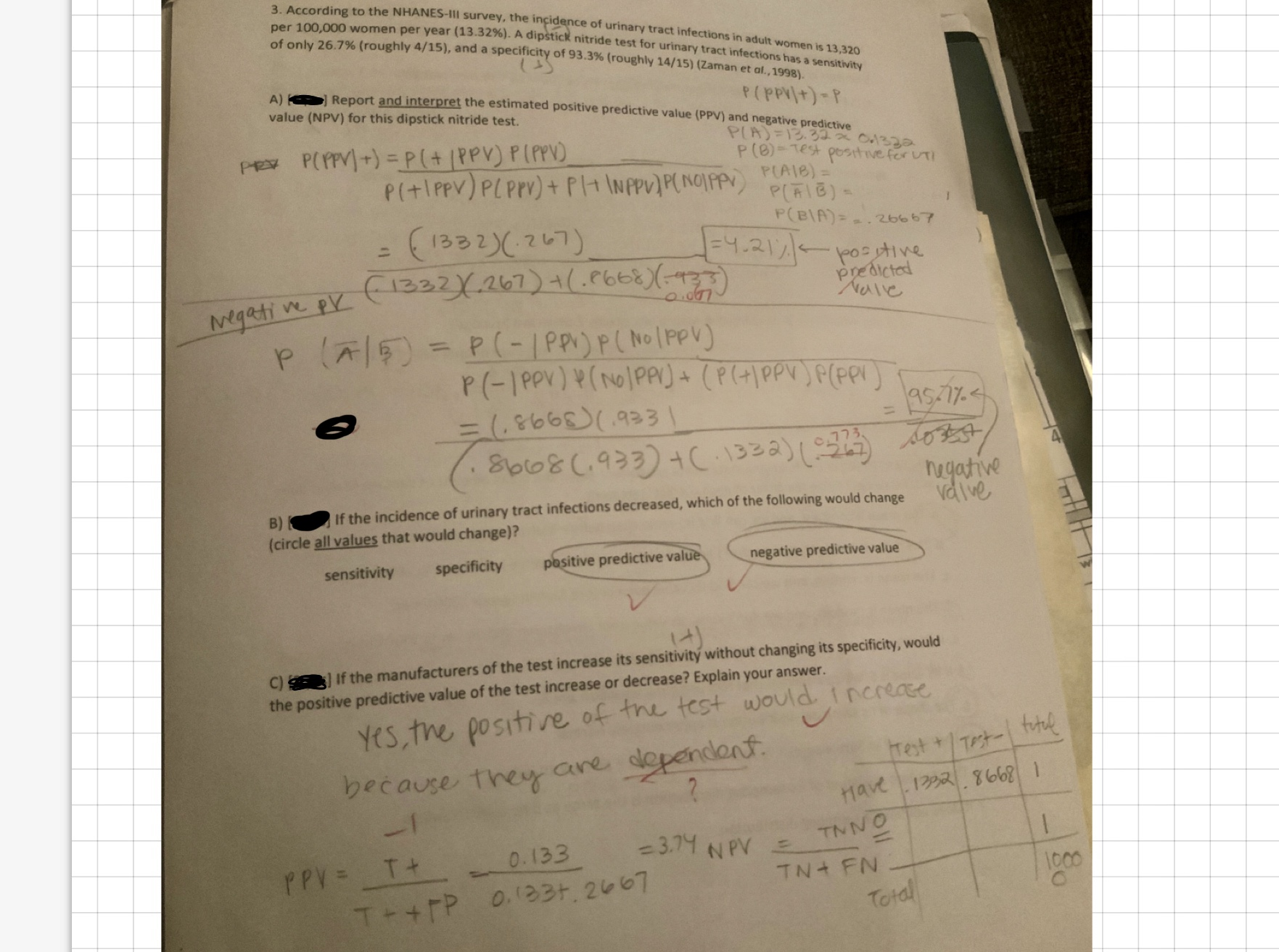# the NHANES-Ill survey, the inçidence of urinary tract infections in adult women is 13,320n per year (13.32%). A dipstick nitride test for urinary tract infections has a sensitivity3. According to NHANES-IIper 100,000 womeof only 26.7% (roughly 4/ 15), and a specificity of 93.3% (roughly 14/15) (zaman et alsurvey, the ingidence of urinary t., 1998).A) Report and interpret the estimated positive predictive value (PPV) and negative predictivevalue (NPV) for this dipstick nitride test.predictedelieMegeti w933nugathveB)If the incidence of urinary tract infections decreased, which of the following would change dive(circle all values that would change)?negative predictive valuesensitivity specificity pasitive predictive valueits sensitivitý without changing its specificity, wouldC)羹the positive predictive value of the test increase or decrease? Explain your answer] If the manufacturers of the test increaseYes the positire of tni test would i nereaetvtrlstbecause they are dgtendontbecause, ther CrePA . %6681 1HaveTN+ FNTot

Question
75 viewshelp_outlineImage Transcriptionclosethe NHANES-Ill survey, the inçidence of urinary tract infections in adult women is 13,320 n per year (13.32%). A dipstick nitride test for urinary tract infections has a sensitivity 3. According to NHANES-II per 100,000 wome of only 26.7% (roughly 4/ 15), and a specificity of 93.3% (roughly 14/15) (zaman et al survey, the ingidence of urinary t ., 1998). A) Report and interpret the estimated positive predictive value (PPV) and negative predictive value (NPV) for this dipstick nitride test. predicted elie Megeti w 933 nugathve B)If the incidence of urinary tract infections decreased, which of the following would change dive (circle all values that would change)? negative predictive value sensitivity specificity pasitive predictive value its sensitivitý without changing its specificity, would C)羹 the positive predictive value of the test increase or decrease? Explain your answer ] If the manufacturers of the test increase Yes the positire of tni test would i nereae tvtrl st because they are dgtendont because, ther Cre PA . %6681 1 Have TN+ FN Tot fullscreen
check_circle

Step 1

Given incidence of urinary tract infections = 13.32%
Nitride test for urinary tract infections sensitivity = 26.7%
NItride test for urinary tract infections specificity = 93.3%

The total women with urinary tract infections among 100,000 women = 13320.
The total women without urinary tract infection among 100,000 women = 86680

The total women with urinary infection and test positive = 13320*0.267 = 3556.44
The total women wth urinary nifection and test negative = 13320 - 3556.44 = 9763.56

The total women without Urinary infection and test negative = 86680*0.933 = 80872.44
The total women without Urinary infection and test positive = 86680 - 80872.44 = 5807.56

All the following calculated values are shown in table form below

Step 2

From the below table
Probability of  being with Urinary tract infection given that test is positive P(With Infection | Test positive) = 3556.44/9364
Probability of being without Urinary tract infection given that test is positive P( Without Infection | Test positive) = 5807.56/9364

Estimated positive predictive value is nothing but the probability of being with Urinary tract infection given that test is positive
P(With Infection | Test positive)  = 3556.44/9364 =  0.379799 = 0.3798 (rounding to four decimal points)

Step 3

From the below table
Probability of  being with Urinary tract infection given that test is negative P(With Infection | Test negative) = 9763.56/90636
Probability of being without Urinary tract infection given that test is negative P( Without Infection | Test negative) = 80872....

### Want to see the full answer?

See Solution

#### Want to see this answer and more?

Solutions are written by subject experts who are available 24/7. Questions are typically answered within 1 hour.*

See Solution
*Response times may vary by subject and question.
Tagged in

### Statistics